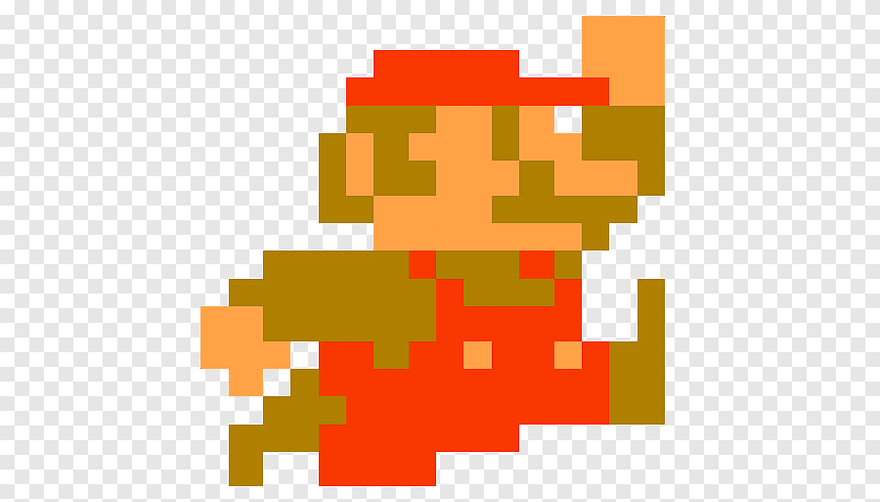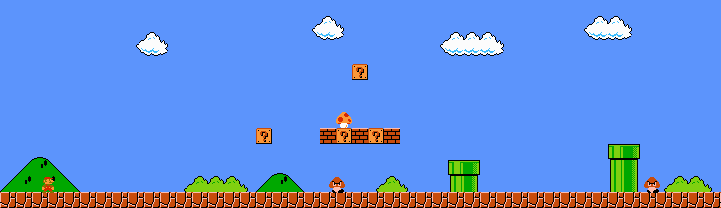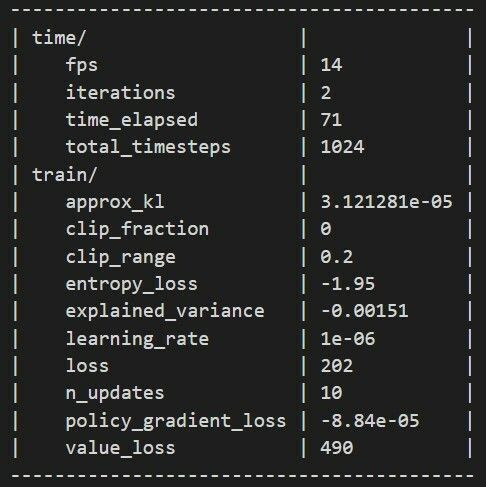# Reinforcement learning in Super Mario bros Pt.2

Before getting here you should setup & preprocess the environment tap your index or thumb finger hovering on me

We are building an AI 🤖 to play 🎮 Super Mario Bros by reinforcement learning method and RL has four key elements.

1. Agent 🕵️Agent can take some action in an environment to have some rewards or penalties.

2. Reward 🏆depending upon agent action he will get a reward or a penalty

3. Environment 🖼️The place where all happens. Agent does specify work according to the AI by analyzing environment and reward or penalty is given by how good or bad does the agent perform in that environment.

The Algorithm we use is PPO Proximal Policy Optimization

## 🚂 Train the model to play

``````import os

from stable_baselines3 import PPO

from stable_baselines3.common.callbacks import BaseCallback
``````

Here we are importing os to work with our file which helps when we save our model every 10000 games or steps so we can have a backup of our progress.

Imported our main Algorithm PPO that will be used to train our AI model or reinforcement learning model

then we imported Base Callback

To save the model 💾

``````class TrainAndLoggingCallback(BaseCallback):

def __init__(self, check_freq, save_path, verbose=1):
super(TrainAndLoggingCallback, self).__init__(verbose)
self.check_freq = check_freq
self.save_path = save_path

def _init_callback(self):
if self.save_path is not None:
os.makedirs(self.save_path, exist_ok=True)

def _on_step(self):
if self.n_calls % self.check_freq == 0:
model_path = os.path.join(self.save_path, 'best_model_{}'.format(self.n_calls))
self.model.save(model_path)

return True
``````

The above step is optional you can skip it if you need. The process happening in the above step is we are saving the trained data at a set of callback intervals. So if we need we don't need to train the model again we can reuse this. Make sure you have enough storage space the model produces a hefty amount of data.

Locate file 📁

``````CHECKPOINT_DIR = './train/'
LOG_DIR = './logs/'
``````

We are specifying where the produced data is located at

Setup Callback 📞🔙

``````callback = TrainAndLoggingCallback(check_freq=10000, save_path=CHECKPOINT_DIR)
``````

This is just the instance of the TrainAndLoggingCallback(). What we are doing here is to save our model every 1000 steps or every 1000 games

We just have it as a backup for future reference else we need to re-run the whole training process.

We are going to setup our PPO model 💃

``````model = PPO('CnnPolicy', env, verbose=1, tensorboard_log=LOG_DIR, learning_rate=0.000001,
n_steps=512)
``````

What we have done is create a variable called `model` and set that to `PPO` which is our model and passing parameters:

1. `CnnPolicy`- It is like a computer-based brain like a Neural Network in deep learning. A bunch of neurons communicate with each other and learn the relationship between different variables. Then there are various policies for different tasks. We used `CnnPolicy` because when it comes to image-based problems this model has its upper hand,
2. `env` - Our environment which we preprocessed.
3. `verbose=1` - This gives us the data when we train the model. like setting it to `0 no output, 1 info, 2 debug`.
4. `tensorboard_log=LOG_DIR` - This helps us to view the metric of how our training is performing as we are running our model.
5. `learning_rate=0.000001` - The learning rate, can be a function of the current progress remaining.
6. `n_steps=512` - The number of steps to run for each environment per update.

The hardest thing in any deep learning or machine learning is Getting the data in the right format.
This one-line code created a temporary AI model.

AI model starts to learn 📖

``````model.learn(total_timesteps=100000, callback=callback)
``````

This is where our AI model starts to learn we are passing some parameters:

1. `total_timesteps=100000` - The total number of samples (env steps) to train on.
2. `callback=callback` - called at every step with the state of the algorithm.

You will get some details about the current process while running `model.learn`.If you see the above then you are good to go else check the previous step or do it as a developer does stackoverflow it.

Let's break down the resulting log by just taking look at some important values to notice.

1. `fps` : Frame Per Second
2. `iterations` : Number of times the process repeated.
3. `time_elapsed` : How long it been training for.
4. `total_timesteps` : How many frames our model goes through.
5. Training Metrics :
• `entropy_loss` (⬇️) : In reinforcement learning, a similar situation can occur if the agent discovers a strategy that results in a reward that is better than it was receiving when it first started, but very far from a strategy that would result in an optimal reward.
• `explained_variance` (⬆️) : The explained variance is used to measure the proportion of the variability of the predictions of a machine learning model. Simply put, it is the difference between the expected value and the predicted value.
• `learning_rate` (📚): It is a tuning parameter in an optimization algorithm that determines the step size at each iteration while moving toward a minimum of a loss function.
• `loss` (⬇️) : loss is the value of the cost function for our training data.
• `value_loss` (⬇️) : val_loss is the value of cost function for our cross-validation data

## Finally ✨

``````model = PPO.load('./train/best_model_1000000')
``````

We are loading the trained model to our algorithm and saying the PPO algorithm to use that specific trained model to play Mario in our case it's `best_model_1000000`.

Ai Plays Mario 🤖

``````state = env.reset()
while True:

action, _ = model.predict(state)
state, reward, done, info = env.step(action)
env.render()
``````

Then we are starting our game and loop through the game. Previously we used some random actions to move Mario now we are using the model.predict(state) to predict according to the model and give certain actions to Mario.

Source Code